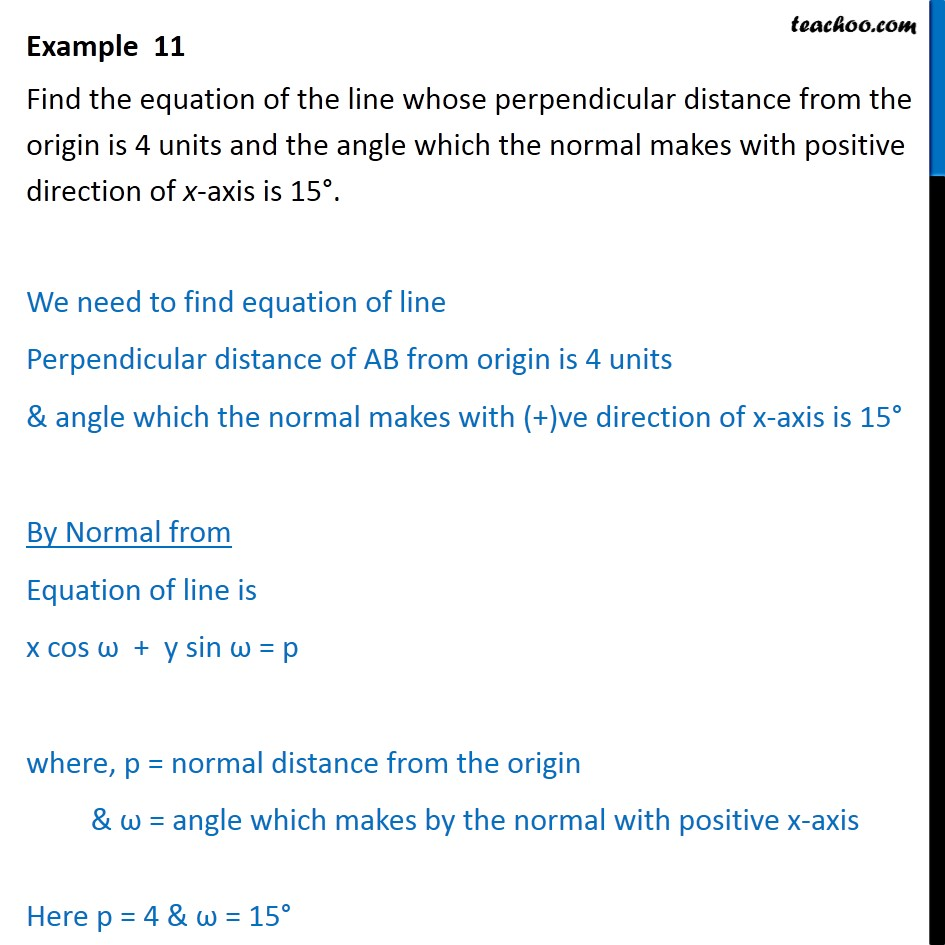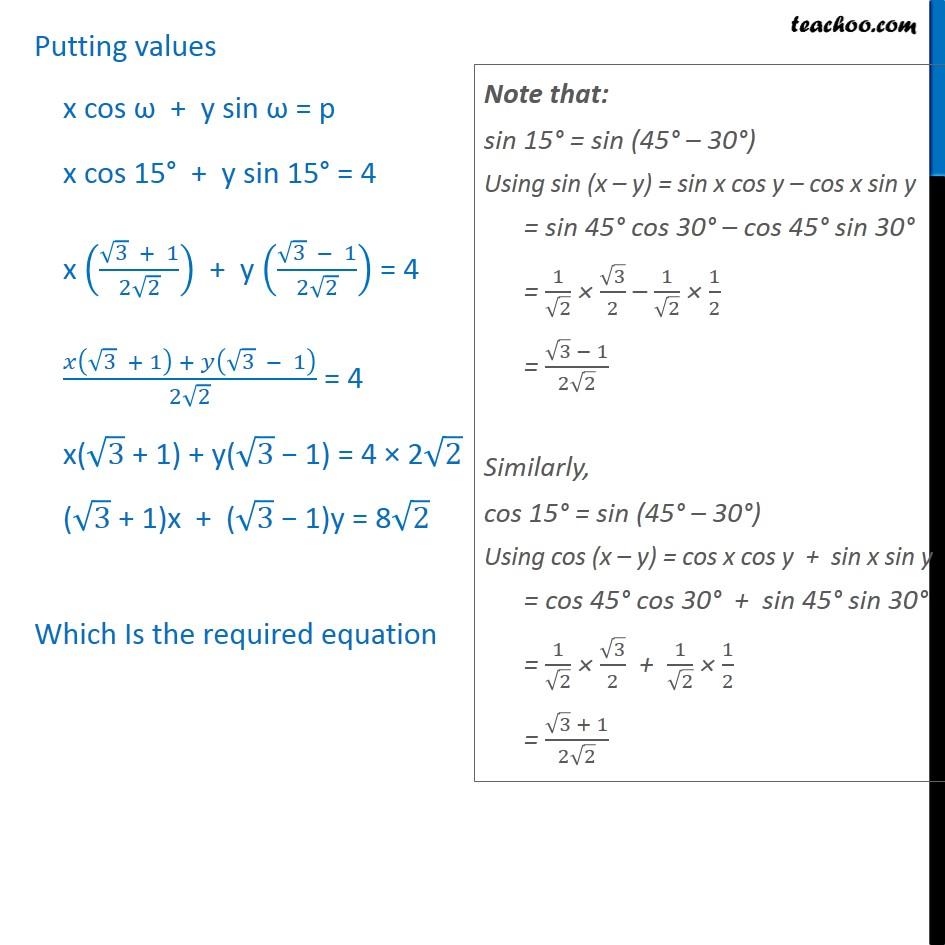Examples

Chapter 9 Class 11 Straight Lines
Serial order wiseLearn in your speed, with individual attention - Teachoo Maths 1-on-1 Class

### Transcript

Example 11 Find the equation of the line whose perpendicular distance from the origin is 4 units and the angle which the normal makes with positive direction of x-axis is 15 . We need to find equation of line Perpendicular distance of AB from origin is 4 units & angle which the normal makes with (+)ve direction of x-axis is 15 By Normal from Equation of line is x cos + y sin = p where, p = normal distance from the origin & = angle which makes by the normal with positive x-axis Here p = 4 & = 15 Putting values x cos + y sin = p x cos 15 + y sin 15 = 4 x (( 3 + 1)/(2 2)) + y (( 3 1)/(2 2)) = 4 ( ( 3 + 1) + ( 3 1))/(2 2) = 4 x( 3 + 1) + y( 3 1) = 4 2 2 ( 3 + 1)x + ( 3 1)y = 8 2 Which Is the required equation